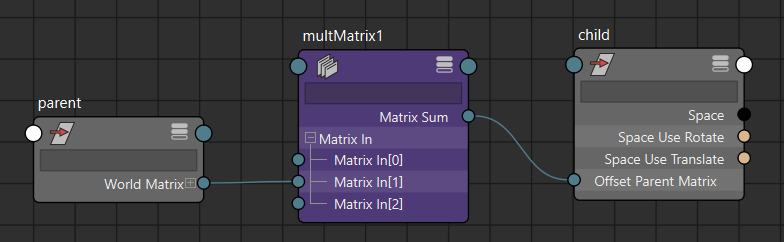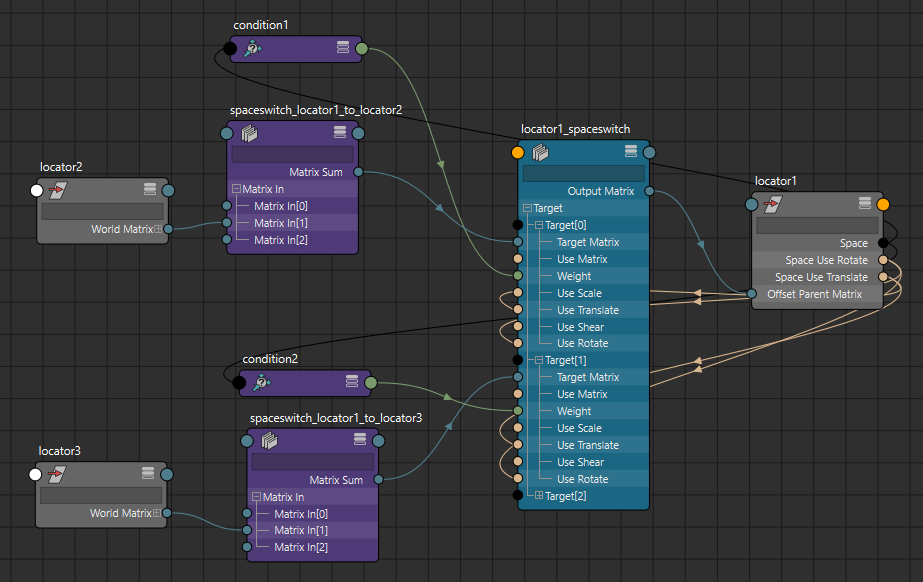# Space Switch Using Offset Parent Matrix

Maya 2020 introduced the offsetParentMatrix attributes to transform nodes. This attribute allows transforms to be driven directly with a matrix and can cut down the number of nodes and connections in your set ups. In this post I'll show how to set up space switching using the offsetParentMatrix attribute instead of constraints and extra transform nodes.

A parent-child relationship is a simple matrix multiplication and can be replicated with a multMatrix node:To maintain the offset when creating the setup, we need to create an offset matrix relative to the parent:

``````offset = (
OpenMaya.MMatrix(cmds.getAttr("{}.worldMatrix".format(child)))
* OpenMaya.MMatrix(cmds.getAttr("{}.matrix".format(child))).inverse()
* OpenMaya.MMatrix(cmds.getAttr("{}.worldInverseMatrix".format(parent)))
)
``````

We also need to remove any existing local transform from the offset so we don't get a double transform when the offsetParentMatrix attribute is connected.

Since the offsetParentMatrix is applied in local space, we need to then multiply the result by the child's parent inverse matrix. However, there seems to be cycle evaluation issues when using a node's parentInverse to calculate the offsetParentMatrix, so you can use the actual parent's world inverse matrix instead. I'm not sure if the parentInverse evaluation issue is a bug or not but we have a work around. This is by design. From Will Telford, Maya Senior Product owner: “The parentMatrix output concatenates the OPM with the parentMatrix. This allows things like existing constraints to continue to function." Here is the multMatrix setup scripted out:

``````mult = cmds.createNode("multMatrix")

offset = matrix_to_list(
OpenMaya.MMatrix(cmds.getAttr("{}.worldMatrix".format(node)))
* OpenMaya.MMatrix(cmds.getAttr("{}.matrix".format(node))).inverse()
* OpenMaya.MMatrix(cmds.getAttr("{}.worldInverseMatrix".format(driver)))
)
cmds.setAttr("{}.matrixIn".format(mult), offset, type="matrix")

cmds.connectAttr("{}.worldMatrix".format(driver), "{}.matrixIn".format(mult))

parent = cmds.listRelatives(node, parent=True, path=True)
if parent:
cmds.connectAttr("{}.worldInverseMatrix".format(parent), "{}.matrixIn".format(mult))

cmds.connectAttr(
"{}.matrixSum".format(mult), "{}.offsetParentMatrix".format(node)
)
``````

To create the space switching, we can connect the output of multiple multMatrix nodes into a blendMatrix node and control the weighting of each target matrix with an enum attribute and conditional nodes to switch between spaces.An added benefit of the blendMatrix node is the ability to disable components of the matrices. This allows you to have a translation-only or rotation-only space switch and even toggle the components on the fly.

To see this whole process scripted out, refer to my Github.